# Concrete Calculator

Use this concrete calculator to estimate how much concrete in volume (cubic ft, cubic yards, or cubic meters), weight or number of bags you would need for your walls, columns, steps, slabs, footings, etc. The calculator uses a standard readymix concrete density by default, but allows for a custom one as well.

Material (optional)

lbs
ft3
lbs/ft3
\$

### Calculation results

0.444 cubic yards (12 ft3) Unit volume Total volume Concrete needed Concrete needed (bags)
Share calculator:

Embed this tool:
get code

## Essential concrete basics for using a concrete calculator

Before using a concrete calculator, it is helpful to understand what concrete is so any estimations you make can be as close to reality as possible. Concrete is a composite material used for all kinds of construction projects, especially where high compressive strength is required. It is composed of fine and coarse aggregate which are bonded via fluid cement paste that hardens over time - usually about a week to reach over 50% of its final strength and a couple of weeks to reach 95% of it. Completely settling in place might take several years, depending on climate and other conditions.

Most concretes in construction are lime-based, such as Portland cement concrete or calcium aluminate cement. Road construction is an exception since in asphalt concrete the cement material is bitumen. Polymer-based concretes are seldom used. Most concrete is embedded with reinforcing materials (e.g. rebar) which provide tensile strength (which concrete lacks on its own) resulting in reinforced concrete.

Different concretes may exhibit highly variable density which is an important part of estimating the amount of concrete you need. For example, Portland cement concrete holds water, but other types of concrete called "Pervious concrete" allow water to pass, making storm drains unnecessary. These two types of conrecte would naturally have vastly different densities. Our concrete calculator allows you to specify custom concrete density for accurate results.

## Calculating how much concrete you need

Most builders face the task of calculating or estimating the amount of concrete needed to build a certain structure: a rectangular or round slab, a wall, supporting square and round columns, staircases and curbs and gutters. The process is as follows:

1. Estimate the volume of concrete needed, using geometrical formulas and the construction plans.
2. Learn the required concrete density, or estimate it based on the chosen concrete mix.
3. Multiply the volume by the density (in the same units)
4. (optional) Estimate the amount of readymix concrete you would need

The final step can be done for a custom mix by calculating the required amounts of gravel, cement and other materials based on the chosen proportions (percentages). Scroll down for more on concrete density and redymix versus custom mix concrete.

Given that there is usually some percentage of concrete lost or wasted during the mixing and pouring process, it is a good idea to consider buying 5-6% more concrete than estimated, to be on the safe side. For example, if our concrete calculator estimates you need 8 tons (~16,000 lbs) of concrete, you need to add at least 5% and purchase a total of 8.4 tons (~16,800 lbs). Use our percentage increase calculator for easier calculation.

### Square or rectangular concrete slab

The volume formula for a rectangular (or square) slab in cubic feet is height(ft) x width(ft) x length(ft), as seen in the figure below: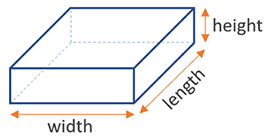For example, 1ft x 3ft x 6ft = 24ft3 (cubic feet) of concrete required. The simples use of our tool is as a concrete slab calculator or concrete wall calculator.

### Concrete wall calculation

For a wall made of concrete the calculation is practically the same: height(ft) x width(ft) x length(ft).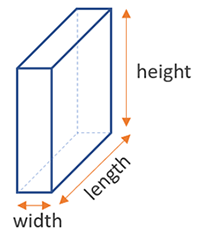For example, to calculate the volume in cubic meters for a wall with a height of 2m, width of 20cm and length of 4m, you need to multiply 2 x 0.2 x 4, which equals 1.6 m3 (cubic meters).

It is no different for a concrete footing, where we again have the familiar formula h x w x l.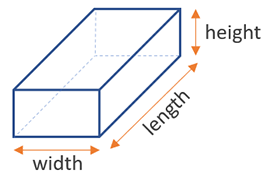### Concrete column

A rectangular column is simple to calculate, except in most cases the height will be much greater than the foundation dimensions, so in our concrete calculator we have defaulted to inches for width and length, and feet for the height (cm and meters respectively).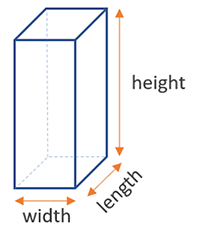Things get more interesting with a round column: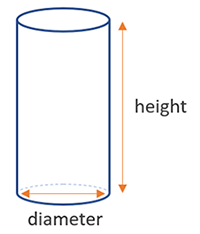If you remember, the volume of a column is its height times the area of its foundation. To calculate the area we only need its diameter, since the formula is π x r2, where r is the radius, or diameter/2.

## Concrete staircase, curb and gutter

More complex concrete forms such as curb and gutter and staircases use the same approach, though the calculation is a bit more complex and so a calculator becomes more essential. For the curb & gutter its just a matter of combining the curb and gutter volume using this formula:

curb depth * (curb height + flag thickness) * length + gutter width * flag thickness * length, where each component is as illustrated below: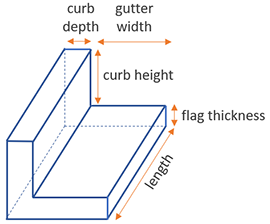A staircase is probably the most complex one, since we need to account for the increasing volume of concrete for each subsequent stair, and also the added depth of the platform on the last step. A calculator like ours is really does a lot of heavy lifting in this case. The volume formula for each particular step is n x step rise x step run x step width, where n is the n-th step. For step 2, with a rise of 6 inches, run of 6 inches and width of 4 feet, the calculation would be 2 x 0.5 x 0.5 x 4 = 2 ft3 (cu ft.)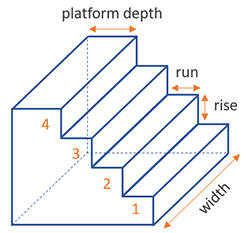Note that larger concrete stairs usually have air space beneath them, thus greatly reducing the amount of concrete you need. They usually have a sloping base, and steps on top. This form is more complex and not currently supported by our calculator.

## Concrete density & common bag sizes

Estimating volume is easy but estimating the required concrete weight (mass) is harder, as we need to know the density of our material, usually given in kg per cubic meter (kg/m3). It is also referred to "density in place". Conventional concrete has density between 1900 kg/m3 and 2400 kg/m3 and can endure, on day 28 of its pouring, and has a compressive strength between 2500 psi and 7000 psi (pounds per square inch) ~ 200-492 kg/cm2. According to the Portland Cement Association concrete with strength between 7000 and 14000 psi is considered high-strength.

Standard U.S. concrete is usually about 2130 kg/m3, which is also the default in this concrete calculator, but you can enter any density in it to customize the calculation. Lightweight concrete is usually below 1500 kg/m3, while electrically conductive concrete is between 1450-1800 kg/m3.

Concrete manufacturers often don't specify the density of their concrete mixture directly, but instead give you the bag yield of the material in place. For example, a conventional redymix concrete bag of 80 lbs may be listed as having a yield of approximately 0.60 cu ft or a 25 kg bag may have a yield of ~0.01 cu m. With a few simple mathematical transformations we can use this number to arrive at the in place density of the material and complete our calculation of the total mass and number of bags of concrete we would need. If you already know the density and volume you can also use our density calculator to complete the calculations.

Common bag sizes in the U.S. are 80 lbs and 60 lbs bags, while 40 lbs are also manufactured by some producers. In Europe the standards are 25 kg and 50 kg bags. All of these are calculated by default in our online tool, depending on your chosen metrics, while you can also specify a custom bag size if you so desire.

## Readymix vs. custom mixed concrete

Our software uses readymix concrete (a.k.a. ready mix concrete) in the calculations it does. Readymix is pre-mixed concrete to which you only need to add water (in proportion as specified by manufacturer). By knowing the pounds or kilograms of concrete you need, you can calculate the amount of cement and other materials you would need for your custom mix. Custom mixing concrete is, however, a complicated matter and we recommend you attempt it only if your knowledge of building and construction materials is on a professional level.

## Concrete in Ancient Rome

Did you know that concrete was known and heavily used in ancient Rome? Romans were so good at making concrete, that some of it is in perfect condition even today, some 2 millennia later. Rather than eroding, like most modern concrete the material seems to gain strength from the exposure, particularly in the presence of sea water.

We are still unable to replicate the concrete of ancient Rome and there are a number of limiting factors which make it a very challenging task. For example - the lack of suitable volcanic rocks - Romans were fortunate the right materials were on their doorstep, almost literally. Another issue is that the Romans didn't follow a precise mixture, so we'll need years of experimenting to arrive at a good approximation.

Modern concrete tunnel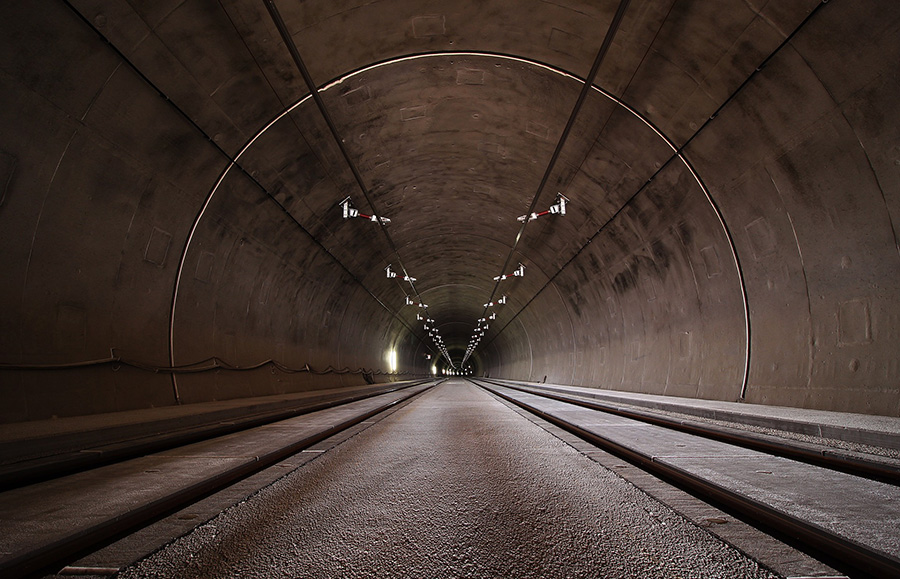If you want your building project to stand the test of time, then a good concrete mixture and estimating the amount of concrete you need are solid first steps.